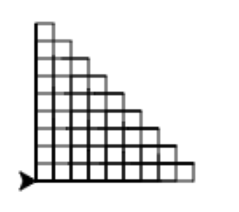# 8.8. Chapter Exercises¶

The code currently draws a square. Change it so that it draws a triangle. Do so by modifying the loop and the turn that the turtle makes without modifying other code.

Remember that to make a complete shape, the turns must sum to 360 degrees.

Turtle procedures

Fix the errors in the code so that it draws an octagon. Do so by fixing the issues in the loop based code. There are three issues to fix.

Remember that to make a complete shape, the turns must sum to 360 degrees.

Turtle procedures

Fix the indention in the code below to correctly draw 20 pentagons (5 sided polygons).

Make sure to use four spaces for each indentation level (the tab key will automatically use 4 spaces).

Turtle procedures

We want to make a series of rectangles such that the first one is 10x90, the next is 20x80, the next is 30x70, … until we draw one that is 90x10. Doing so will make a “staircase grid” like the one shown below:Below is a program with rectangle already defined. Add code that uses a for loop to call the procedure with the correct values for its parameters. Make sure that the 90 width and 10 height rectangle is the first one you draw. You should only need one loop - it must count down from 90 to 10.

Start by writing a loop that just prints out the values 90, 80, 70, …, 10. Then you can modify the code to use those values to draw rectangles of the appropriate widths and heights.

Hint: The values we use for width and height will always add to 100. So if we call one of them $$w$$, the other must be $$100 - w$$.

The autograder will not verify everything about your program. It is up to you to determine if your program is correct or not.

Turtle procedures